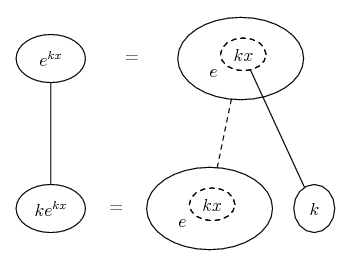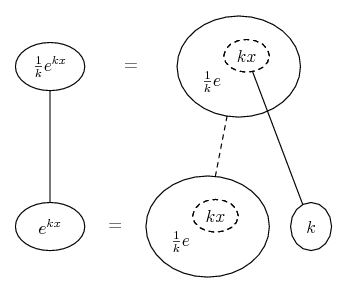# Thread: e^x derivative?

1. ## e^x derivative?

Hi everyone! looking for some basics,

The derivative of e^x = e^x

but what is e^3x? is it e^3x?

2.Originally Posted by NFZ17Hi everyone! looking for some basics,

The derivative of e^x = e^x

but what is e^3x? is it e^3x?
If $\displaystyle y = e^{kx}$ then $\displaystyle \frac{dy}{dx} = k e^{kx}$. This follows directly from the chain rule and your correctly stated result that the derivative of $\displaystyle e^x$ is $\displaystyle e^x$.

3. So what if

dy/dx=e^kx

What is y? do I just integrate? e^kx+1/kx+1 ?

4.Originally Posted by NFZ17So what if

dy/dx=e^kx

What is y? do I just integrate? e^kx+1/kx+1 ?
It should be clear from my first post that if $\displaystyle \frac{dy}{dx} = e^{kx}$ then $\displaystyle y = \frac{1}{k} e^{kx} + C$.

5. Just in case a picture helps..._________________________________________

Don't integrate - balloontegrate!

Balloon Calculus; standard integrals, derivatives and methods

Balloon Calculus Drawing with LaTeX and Asymptote!

#### Search Tags

derivative